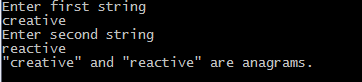## Print this Job Post# Anagram in c

Anagram in c: c program to check whether two strings are anagrams or not, string is assumed to consist of alphabets only. Two words are said to be anagrams of each other if the letters from one word can be rearranged to form the other word. From the above definition it is clear that two strings are anagrams if all characters in both strings occur same number of times. For example "abc" and "cab" are anagram strings, here every character 'a', 'b' and 'c' occur only one time in both strings. Our algorithm tries to find how many times characters appear in the strings and then comparing their corresponding counts.

## C anagram programming code

#include <stdio.h>
#include<conio.h>
int check_anagram(char [], char []);
int main()
{
char a, b;
int flag;
printf("Enter first string\n");
gets(a);
printf("Enter second string\n");
gets(b);
flag = check_anagram(a, b);
if (flag == 1)
printf("\"%s\" and \"%s\" are anagrams.\n", a, b);
else
printf("\"%s\" and \"%s\" are not anagrams.\n", a, b);
getch();
}
int check_anagram(char a[], char b[])
{
int first = {0}, second = {0}, c = 0;
while (a[c] != '\0')
{
first[a[c]-'a']++;
c++;
}
c = 0;
while (b[c] != '\0')
{
second[b[c]-'a']++;
c++;
}
for (c = 0; c < 26; c++)
{
if (first[c] != second[c])
return 0;
}
return 1;
}

## Output of program: